×
Get Full Access to Statistics For Business And Economics - 12 Edition - Chapter 8 - Problem 27e
Get Full Access to Statistics For Business And Economics - 12 Edition - Chapter 8 - Problem 27e

×

# A paired difference experiment yielded nd pairs of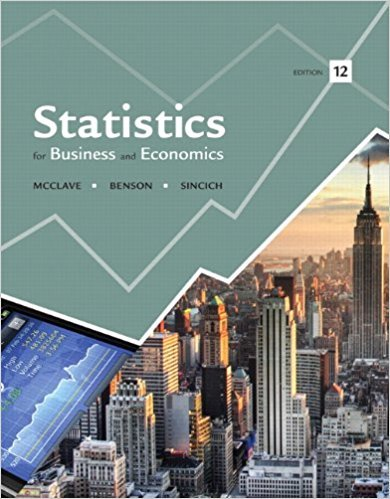ISBN: 9780321826237 51

## Solution for problem 27E Chapter 8

Statistics for Business and Economics | 12th Edition

• Textbook Solutions
• 2901 Step-by-step solutions solved by professors and subject experts
• Get 24/7 help from StudySoup virtual teaching assistantsStatistics for Business and Economics | 12th Edition

4 5 1 257 Reviews
24
2
Problem 27E

Problem 27E

A paired difference experiment yielded nd pairs of observations. In each case, what is the rejection region for testing H0 : µd > 2?

a. n d = 12, α = .05

b. n d = 24, α = .10

c. n d = 4, α = .025

d. n d = 80, α = .01

Step-by-Step Solution:

Problem 27E

A paired difference experiment yielded nd pairs of observations. In each case, what is the rejection region for testing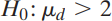?

a.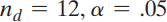b.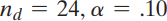c.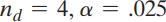d.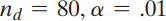Step by Step Solution

Step 1 of 5

Given,At significance levelwe will reject , whereis based on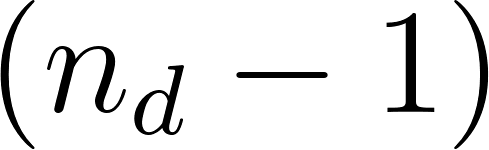degrees of freedom.Step 2 of 5

Step 3 of 5

##### ISBN: 9780321826237

Unlock Textbook Solution